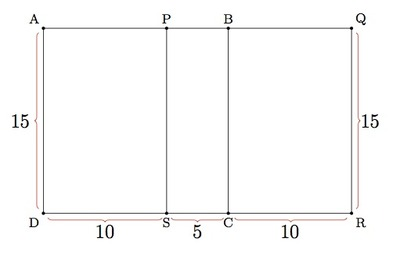# Overlapping Squares

Alignments to Content Standards: 6.RP.A.3.c

Two congruent squares, $ABCD$ and $PQRS$, have side length 15. They overlap to form the 15 by 25 rectangle $AQRD$ shown. What percent of the area of rectangle $AQRD$ is shaded?## IM Commentary

This problem provides an interesting geometric context to work on the notion of percent. Two different methods for analyzing the geometry are provided: the first places the two squares next to one another and then moves one so that they overlap. The second solution sets up an equation to find the overlap in terms of given information which reflects the mathematical ideas described in cluster 6.EE.B, Reason about and solve one-variable equations and inequalities.

Note that the geometric intuition that supports students here begins in Kindergarten and first grade:

K.G.6 Compose simple shapes to form larger shapes.
1.G.2 Compose two-dimensional shapes (rectangles, squares, trapezoids, triangles, half-circles, and quarter-circles) or three-dimensional shapes (cubes, right rectangular prisms, right circular cones, and right circular cylinders) to create a composite shape, and compose new shapes from the composite shape.

and is built on in 3rd and 4th grade:

3.MD.7 Relate area to the operations of multiplication and addition.
4.MD.3 Apply the area and perimeter formulas for rectangles in real world and mathematical problems.

Without the requirement to express the area of the shaded rectangle as a percent of the square, this could be a high-level 4th grade task.

Note that "calculating a percent given a part and a whole" is not explicitly mentioned in standard 6.RP.A.3c; however, that particular problem formulation fits well with the named sixth grade percent work, and including it in sixth grade is a reasonable instructional decision.

This task was adapted from problem #13 on the 2011 American Mathematics Competition (AMC) 8 Test. The responses to multiple choice answers for the problem had the following distribution:

 Choice Answer Percentage of Answers (A) 15 17.84 (B) 18 14.26 (C)* 20 48.92 (D) 24 5.70 (E) 25 9.71 Omit - 3.51

Of the 153,485 students who participated, 72,648 or 47% were in 8th grade, 50,433 or 33% were in 7th grade, and the remainder were less than 7th grade.

## Solutions

Solution: Overlapping the squares

If we put two 15 by 15 squares next to one another so that they share one side, then we get a 15 by 30 rectangle as pictured below:We get the 15 by 25 rectangle in the problem by moving square 1 to the left by 5 units. This is pictured below with the vertices of the new squares labelled as in the original picture.To find the percentage of area shaded, notice that $\overline{SC}$ and $\overline{DR}$ are the bases for rectangles $PBCS$ and $AQRD$ (respectively) and that these rectangles have the same height of 15. So the fraction of the rectangle that is shaded is: $$\frac{15\cdot\text{length}(\overline{SC})}{15\cdot\text{length}(\overline{DR})} = \frac{15\cdot 5}{15\cdot 25}=\frac{5}{25}.$$ Thus, $\frac{5}{25}$ of the total area is shaded. We want to know what percent of the area is shaded which means that we want to express the fraction $\frac{5}{25}$ with a denominator of $100$. Multiplying numerator and denominator by $4$ gives $$\frac{5}{25} = \frac{4 \times 5}{4 \times 25} = \frac{20}{100}.$$ This means that 20% of the area is shaded.

Solution: Representing the unknown with an equation and solving (6.EE.B)

We are given that the length of $\overline{DC}$ and $\overline{SR}$ are both fifteen and that the length of $\overline{DR}$ is 25. We can rewrite the length of $\overline{DR}$ as follows: $$\text{length}(\overline{DR}) = \text{length}(\overline{DC}) + \text{length}(\overline{SR}) - \text{length}(\overline{SC})$$ because segments $\overline{DC}$ and $\overline{SR}$ make up segment $\overline{DR}$ but they overlap in segment $\overline{SC}$. Plugging in the information that has been given into this equation we find $$25 = 15 + 15 - \text{length}(\overline{SC}).$$ We can add $\text{length}(\overline{SC})$ to both sides of this equation and subtract 25 from both sides to find $$\text{length}(\overline{SC}) = 5.$$

The remainder of the argument is the same as in the previous solution: to find the percentage of area shaded, notice that $\overline{SC}$ and $\overline{DR}$ are the bases for rectangles $PBCS$ and $AQRD$ (respectively) and that these rectangles have the same height of 15. So the fraction of the rectangle that is shaded is: $$\frac{15\cdot\text{length}(\overline{SC})}{15\cdot\text{length}(\overline{DR})} = \frac{15\cdot 5}{15\cdot 25}=\frac{5}{25}.$$ Thus, $\frac{5}{25}$ of the total area is shaded. We want to know what percent of the area is shaded which means that we want to express the fraction $\frac{5}{25}$ with a denominator of $100$. Multiplying numerator and denominator by $4$ gives $$\frac{5}{25} = \frac{4 \times 5}{4 \times 25} = \frac{20}{100}.$$ This means that 20% of the area is shaded.